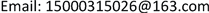1. 引言

2. 卷积神经网络的卷积核传递

C = 1 2 ∑ j ( y j − a j ) 2 (1)

y j 为输出层第j个神经元的实际值， a j 为第j个神经元的理想值，采用方程(1)的形式定义目标函数，方便后面的求导计算：

∂ C ∂ a j = ( a j − y j ) (2)

δ j L = ∂ C ∂ z j L = ∂ C ∂ a j L ∂ a j L ∂ z j L (3)

z j L 代表第L层，即输出层第j个神经元， a j L 为神经元 z j L 通过激活函数 f ( z ) 后的激活值，那么即可求出输出层第j个神经元的误差为：

δ j L = ( a j − y j ) f ′ ( z ) (4)

δ j l = ∂ C ∂ z j l = ∑ k ∂ C ∂ z k l + 1 ∂ z k l + 1 ∂ z j l = ∑ k ∂ z k l + 1 ∂ z j l δ k l + 1 (5)

z k l + 1 = ∑ j ω k j l + 1 a j l + b k l + 1 = ∑ j ω k j l + 1 f ( z j l ) + b k l + 1 (6)

∂ z k l + 1 ∂ z j l = w k j l + 1 f ′ ( z j l ) (7)

δ j l = ∑ k ω k j l + 1 δ k l + 1 f ′ ( z j l ) (8)

∂ C ∂ ω l = ∂ C ∂ z l ∂ z l ∂ ω l = δ l ( a l − 1 ) T (9)

∂ C ∂ b l = ∂ C ∂ z l ∂ z l ∂ b l = δ l (10)

δ j l = u p s a m p l e ( δ j l + 1 ) ⊗ f ′ ( z j l + 1 ) (11)

for j = 1 : numel(net.layers{l}.a)

net.layers{l}.d{j} = net.layers{l}.a{j} .* (1 - net.layers{l}.a{j}) .* (expand(net.layers{l + 1}.d{j}, [net.layers{l + 1}.scale net.layers{l + 1}.scale 1]) / net.layers{l + 1}.scale ^ 2);

end

net.layers{l}.a为第1层神经元，numel(net.layers{l}.a为卷积层神经元的个数，这里net.layers{l}.a{j} .* (1 - net.layers{l}.a{j})是对激活函数f(z)求导。expand(net.layers{l + 1}.d{j}, [net.layers{l + 1}.scale net.layers{l + 1}.scale 1]) / net.layers{l + 1}.scale ^ 2是上采样操作。

δ j l = ∑ k ( δ k l + 1 ⊗ r o t 180 ( ω j k ) ) (12)

for i = 1 : numel(net.layers{l}.a)

z= zeros(size(net.layers{l}.a{1}));

for j = 1 : numel(net.layers{l + 1}.a)

z = z + convn(net.layers{l + 1}.d{j}, rot180(net.layers{l + 1}.k{i}{j}), 'full');

end

net.layers{l}.d{i} = z;

end

numel(net.layers{l}.a)为池化层神经元的个数，numel(net.layers{l + 1}.a)为池化层下一层即卷积层神经元的个数，net.layers{l}.d{i}为池化层第1层第i个神经元的误差。

∂ C ∂ ω j k l = ∂ C ∂ z j l ∂ z j l ∂ ω j k l = δ l * r o t ( a k l − 1 ) (13)

∂ C ∂ b j l = ∑ δ j l / s i z e ( δ j l ) (14)

for j = 1 : numel(net.layers{l}.a)

for i = 1 : numel(net.layers{l - 1}.a)

net.layers{l}.dk{i}{j}=convn(flipall(net.layers{l-1}.a{i}),net.layers{l}.d{j},'valid')/size(net.layers{l}.d{j}, 3);

net.layers{l}.db{j} = sum(net.layers{l}.d{j}(:)) / size(net.layers{l}.d{j}, 3);

end

numel(net.layers{l}.a 为卷积层l神经元的个数，net.layers{l}.dk{i}{j}为第l层卷积核k{i}{j}的误差。net.layers{l}.db{j}为卷积层偏置的误差。

3. 深度学习算法优化

f ( x ) = 2 1 + e − 2 x − 1 (15)

Tanh激活函数在求导计算时，涉及指数运算，在处理大规模数据输入时，时间代价较大，为了更加快速地进行误差传递，采用 f ( x ) = max ( 0 , x ) 即Relu函数激活   ，该激活函数及其导数的图像如图8所示。从图中可以看出，当输入大于0时，其梯度快速增加，解决梯度消失问题，提供神经网络稀疏表达能力，但是当输入小于0时，梯度为0，在训练过程中权值无法更新，造成部分神经元死亡。为了解决这一问题，需要对max(0, x)激活函数进行改进。为了避免梯度消失的情况出现，将激活函数小于0范围内，增加一个修正系数α，即： f ( x ) = α x , x < 0 。既修正了数据分布，又保留了一些负轴的值，使得负轴信息不会全部丢失，但是修正系数α的选择无法直接确定。为了获得合适的修正系数，对于特定的神经网络，设置同样的修正系数进行训练。图9是采用不同的修正系数来训练同一个神经网络的输出结果。α = 0的情况即为max(0,x)激活函数的训练情况，其误差函数下降速度最缓，部分神经元已经死亡。增加修正系数后，误差函数下降明显提速，说明修正系数有效避免激活函数梯度消失的区间。为了获得更优的修正系数，继续增加修正系数的值，进行训练，从训练结果来看，当系数增加到0.06，误差函数的下降梯度变缓，因此，通过6组实验数据的训练曲线来看，修正系数α取0.05时，训练效果最佳。

4. 总结